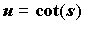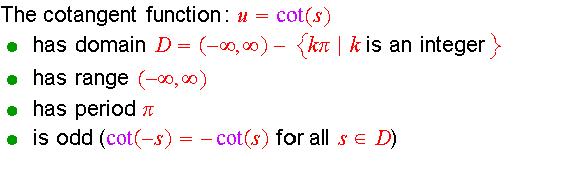# The Trigonometric (Circular) Functions in Radians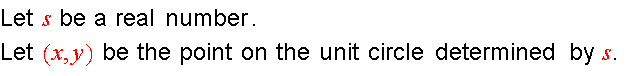Exercise 1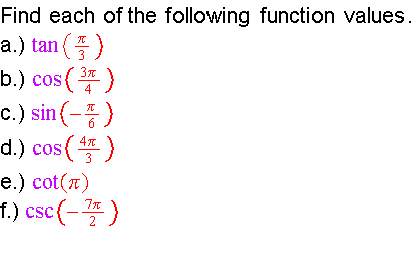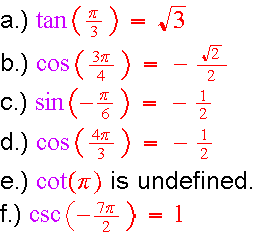Exercise 2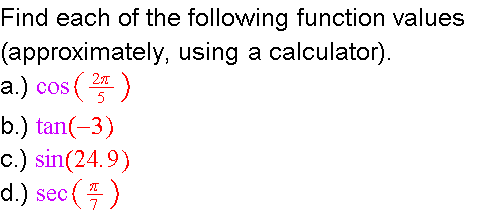The Sine Function

Sketch the graph of y=sin(s)  by plotting points.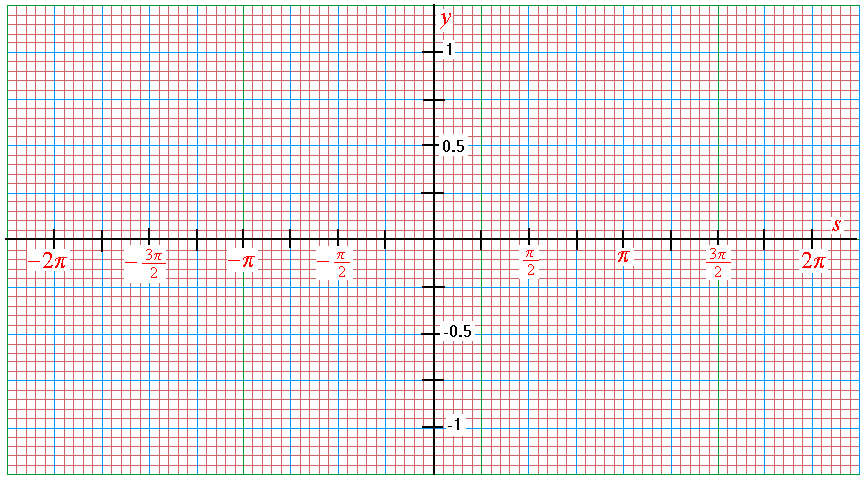Graph of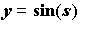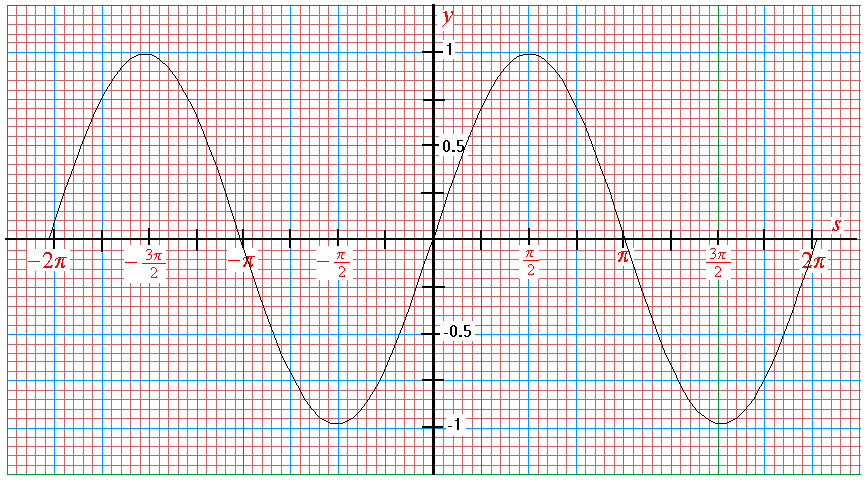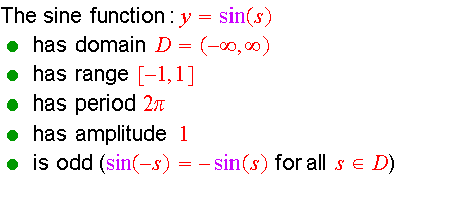The Cosine Function

Sketch the graph of y=cos(s)  by plotting points.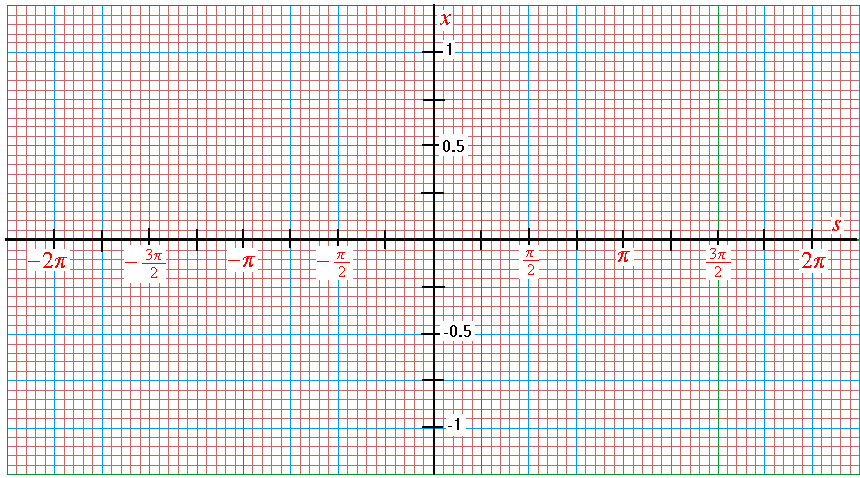Graph of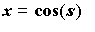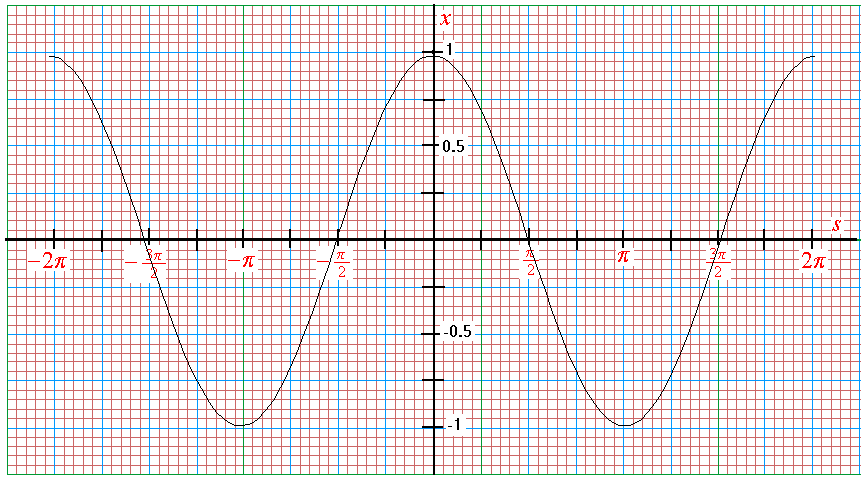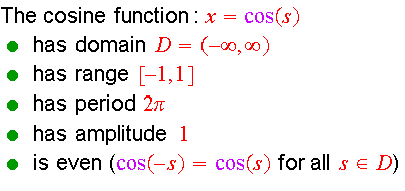The Cosecant Function

Sketch the graph of u =csc(s)  by plotting points.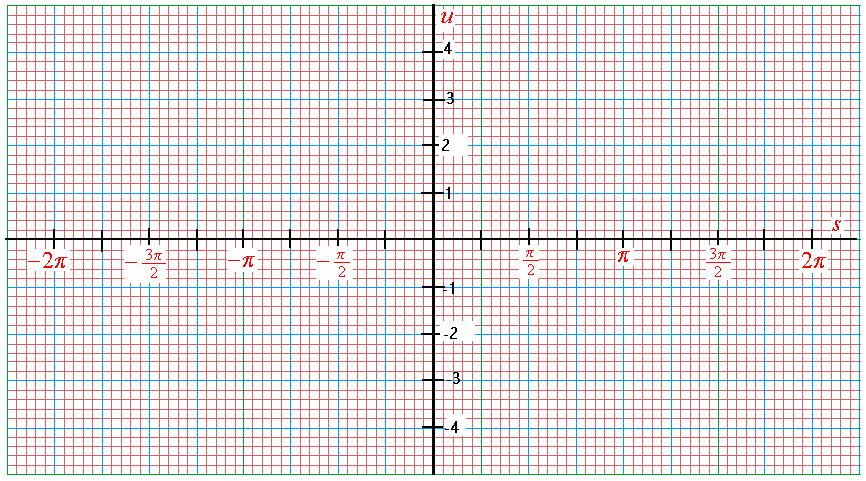Graph of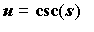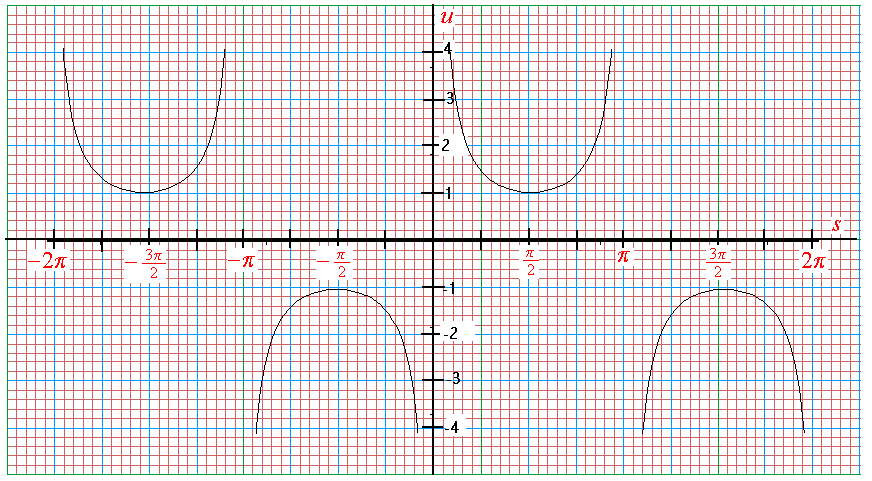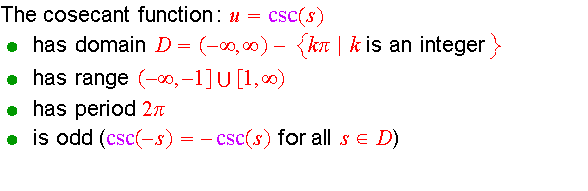The Secant Function

Sketch the graph of u =sec(s)  by plotting points.Graph of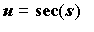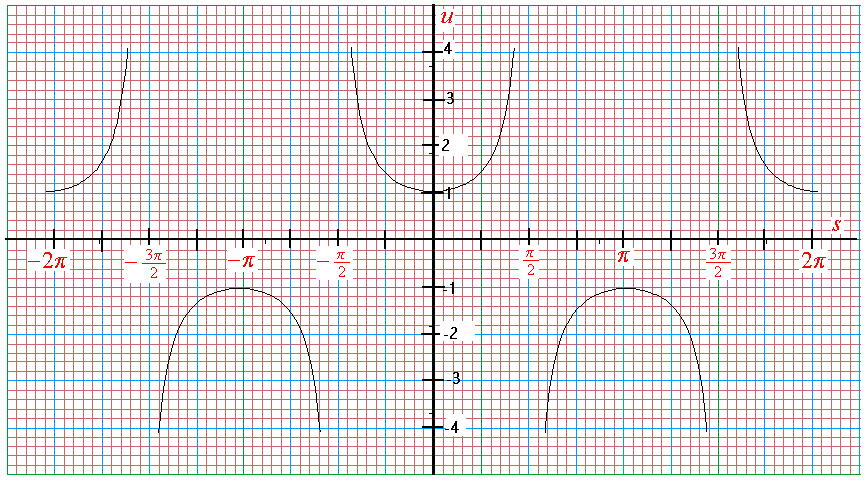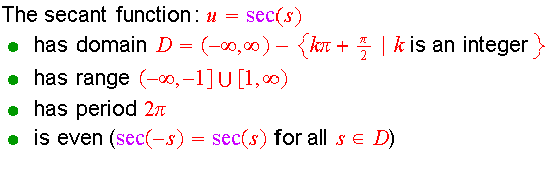The Tangent Function

Sketch the graph of u =tan(s)  by plotting points.Graph of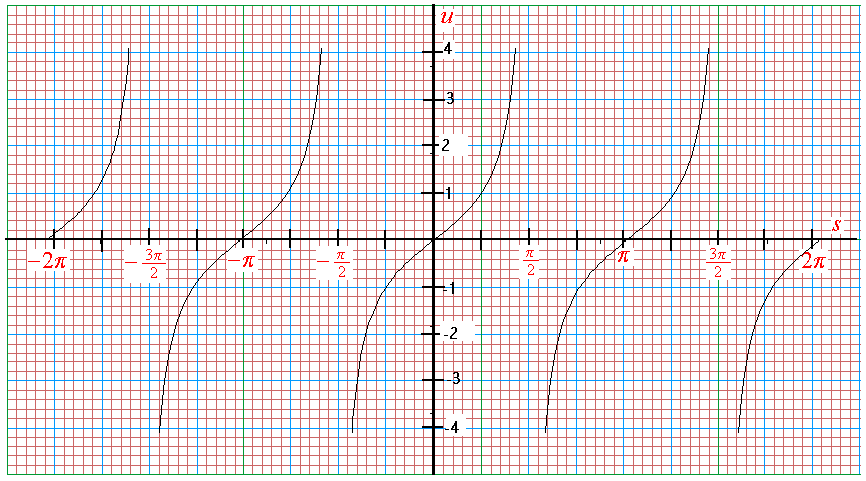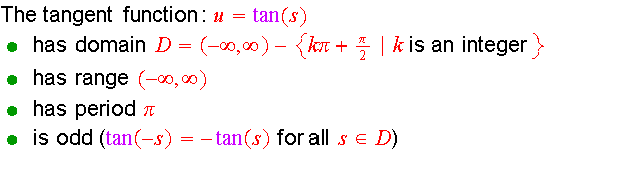The Cotangent Function

Sketch the graph of u =cot(s)  by plotting points.Graph of